# Is A Polynomial A Function? (7 Common Questions Answered)

Polynomials and functions come up all the time in mathematics (specifically in algebra), so it helps to know how these two ideas are related.

So, is a polynomial a function?  A polynomial is a function since it passes the vertical line test: for an input x, there is only one output y. Polynomial functions are not always injective (some fail the horizontal line test). A polynomial function has the form P(x) = anxn + …+ a1x + a0, where a0, a1,…, an are real numbers.

Of course, every polynomial is a function, but not every function is a polynomial.  There are also trigonometric function, power functions, and radical functions that are not polynomials.

In this article, we’ll talk about the connection between polynomials and functions.  We’ll also look at plenty of examples to make the concepts clear.

Let’s get started.

## Is A Polynomial A Function?

A polynomial function is a specific type of function.  A polynomial function has the form

• P(x) = anxn + an-1xn-1 + … + a1x + a0

where a0, a1, … , an are real numbers.

Note that every polynomial is a function, but not every function is a polynomial.  For example, the following functions are not polynomial functions:

• f(x) = sin(x)
• g(x) = 2x
• h(x) = √x

The last example is important to note.  Remember that we can rewrite √x as x1/2.  However, this is not a polynomial, since x is raised to a power (1/2) that is not an integer.

### How To Determine If A Function Is A Polynomial

To determine if a function is a polynomial, examine each term of the function.  Each term should have the form akxk where k is a nonnegative integer and ak is a real number.

For a polynomial, all of the powers of x in any term must be nonnegative integers (positive numbers with no fractions or decimals).  So, the powers of x in a polynomials can be 0, 1, 2, 3, 4, …

So, a function is not a polynomial function if it has:

• powers of x that are fractions or decimals (not whole numbers)
• powers of x that are negative
• terms that are not of the form akxk, such as sin(x) (a trig function) or 2x (an exponential function)

Let’s look at some examples to make the concept clear.

#### Example 1: A Function That Is A Polynomial Function

Consider the function f(x) = 2x3 – 5x + 4.

This is a polynomial function since:

• Every power of x is a nonnegative integer (the power of x is zero for the constant term 4).
• There are no negative powers of x
• Every term is of the form akxk

You can see the graph of this function below.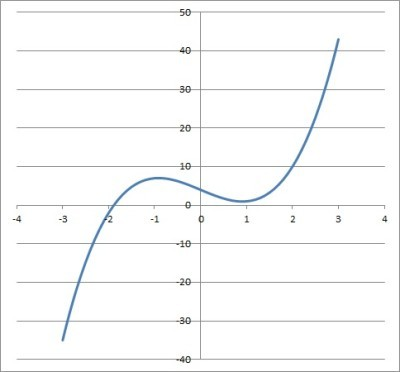The graph of the cubic function f(x) = 2x3 – 5x + 4. This is a polynomial function.

#### Example 2: A Function That Is A Polynomial Function

Consider the function f(x) = (x – 2)(x – 4).

We can use FOIL and combine like terms to get:

• f(x) = (x – 2)(x – 4)
• f(x) = x2 – 4x – 2x + 8  [FOIL]
• f(x) = x2 – 6x + 8  [combine like terms]

This is a polynomial function since:

• Every power of x is a nonnegative integer (the power of x is zero for the constant term 8).
• There are no negative powers of x
• Every term is of the form akxk

You can see the graph of this function below.

#### Example 3: A Function That Is Not A Polynomial Function

Consider the function f(x) = 3x + √x.

This is not a polynomial function since:

• The power of x in the second term is ½, which is not an integer (remember √x = x1/2).

You can see the graph of this function below.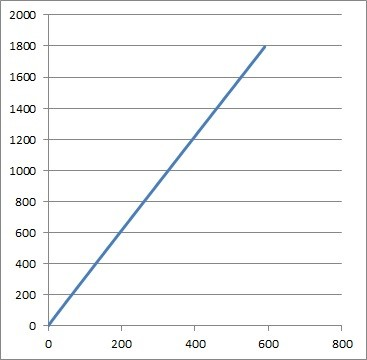The graph of the function f(x) = 3x + √x looks linear, but it is not: it has a slight curve to it. This is not a polynomial function.

#### Example 4: A Function That Is Not A Polynomial Function

Consider the function f(x) = 1 / x.

This is not a polynomial function since:

• The power of x in the only term is -1, which is a negative power (remember 1/x = x-1).

You can see the graph of this function below.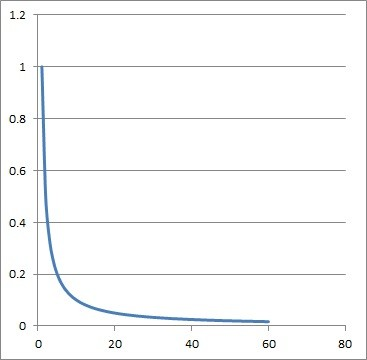The graph of the function f(x) = 1/x has a discontinuity at x = 0. This is not a polynomial function (but it is a rational function).

#### Example 5: A Function That Is Not A Polynomial Function

Consider the function f(x) = 2x + 9

This is not a polynomial function since:

• The first term is not of the form akxk  (2x is a power function).

You can see the graph of this function below.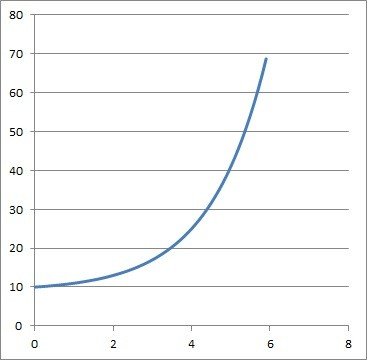The graph of the function f(x) = 2x + 9 is concave up (convex). This is not a polynomial function.

### Is A Polynomial A Rational Function?

Every polynomial function is a rational function.  Remember that a rational function R(x) has the form

• R(x) = P(x) / Q(x)

where P(x) and Q(x) are both polynomial functions.  If we take Q(x) = 1 (which is a polynomial), we get the rational function

• R(x) = P(x) / 1
• R(x) = P(x)

So, every polynomial function is a rational function.  Note: if P(x) has real coefficients and we take Q(x) to be any real constant, then R(x) = P(x) / Q(x) will be a polynomial.

However, the converse is not true.  There are plenty of rational functions that are not polynomial functions.

You can learn how to find the domain and range of a polynomial here.

#### Example: A Rational Function That Is Not A Polynomial Function

Consider the function R(x) = (x + 3) / (x2 + 1)

R(x) is a rational function with P(x) = x + 3 and Q(x) = x2 + 1.  However, R(x) is not a polynomial function, since its denominator Q(x) = x2 + 1 is not a real constant.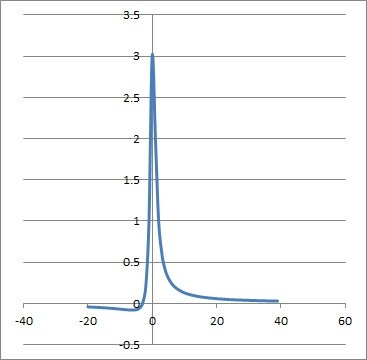The graph of the rational function R(x) = (x + 3) / (x2 + 1). It has a horizontal asymptote at y = 0.

### Is A Polynomial A Linear Function?

A polynomial may be a linear function in some cases.  However, not every polynomial function is a linear function.

It is true that every linear function is a polynomial function, since a linear function f(x) has the form

• f(x) = ax + b

where a and b are real numbers.

#### Example 1: A Polynomial Function That Is A Linear Function

Consider the polynomial function f(x) = 2x + 8.

This is a linear function, since it has the form ax + b (with a = 2 and b = 8).

#### Example 2: A Polynomial Function That Is Not A Linear Function

Consider the polynomial function f(x) = 11x2 + 2x – 1.

This is not a linear function, since it has a quadratic term 11x2.  This makes it a quadratic function, not a linear function.

### Is A Polynomial A Power Function?

A polynomial is a power function in some cases (specifically, for a monomial, when there is only one term in the polynomial).  More generally, a polynomial function is a sum of power functions.  Remember that:

• A power function has the form f(x) = akxk, where ak is a real number and k is a nonnegative integer.
• A polynomial has the form P(x) = anxn + … + a1x + a0,where all of the ai coefficients are real numbers and the powers of x are all nonnegative integers.

So the following are power functions:

• f(x) = 5x3
• g(x) = 8x2
• h(x) = 4x

while their sum is a polynomial function:

• P(x) = f(x) + g(x) + h(x)
• =5x3 + 8x2 + 4x

### Is A Polynomial Function Always Continuous?

A polynomial function is always continuous.  This is because:

• A polynomial function cannot have horizontal asymptotes.  As the input x approaches positive or negative infinity, the output f(x) increases or decreases without bound (meaning there is no “boundary line” or horizontal asymptote).
• A polynomial function cannot have vertical asymptotes.  Since there is no denominator, there is no way to “divide by zero” for such a function (on the other hand, a rational function may have vertical asymptotes).

In the case of piecewise functions whose parts are polynomial functions, we can have holes (removable discontinuities) or “jump” discontinuities.

#### Example 1: A Piecewise Function With A Hole (Removable Discontinuity)

Consider the piecewise function defined by:

• f(x) = 2x for x < 0 and x2 for x > 0

Note that each part of the function is a polynomial function.

This function is continuous everywhere except at x = 0.  The reason is that the function is not defined for x = 0.

We could make the function continuous by adding “f(x) = 0 for x = 0” to the function definition.  This would make the function continuous at x = 0, since the left and right hand limits are both 0 as x approaches zero.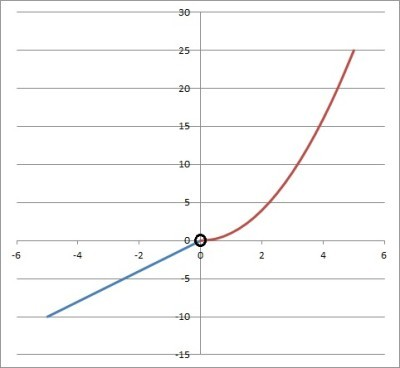Both parts of this piecewise function are polynomials, but the function has a discontinuity at x = 0, where the function is not defined.

#### Example 2: A Piecewise Function With A ”Jump” Discontinuity

Consider the piecewise function defined by

• f(x) = x for x < 0 and x + 1 for x >= 0.

Note that each part of the function is a polynomial function.

This function is continuous everywhere except at x = 0.  The reason is that the function’s left and right hand limits do not agree at x = 0.

The left hand limit is 0 at x = 0, while the right hand limit is 1 at x = 0.  Since the limits do not agree at x = 0, we have a jump discontinuity.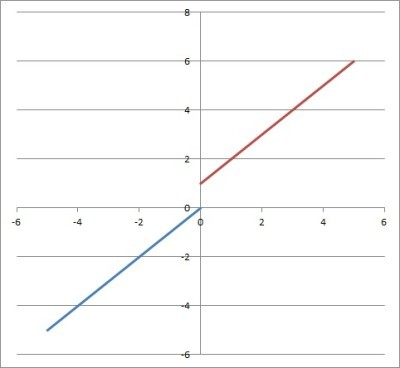Both parts of this piecewise function are polynomials, but the function has a jump discontinuity at x = 0, where the left and right hand limits do not agree.

### What Is A Root Of A Polynomial Function?

A root of a polynomial function is an input (x-value) that gives an output (y-value) of zero.  That is:

• if we have a polynomial function P(x), then x = a is a zero of f(x) if and only if P(a) = 0.

Note that this also means that (x – a) is a linear factor of P(x).  That is, we can write

• P(x) = (x – a)Q(x)

for some polynomial function Q(x) (note that Q(x) could be constant).  This tells us that P(a) is zero, since:

• P(x) = (x – a)Q(x)
• P(a) = (a – a)Q(a)  [substitute x = a]
• P(a) = 0*(Q(0))  [a – a = 0]
• P(a) = 0

A root of a polynomial is also called a zero. You can learn more about the zeros of functions in my article here.

## Conclusion

Now you know about how polynomials and functions are connected. You also know how to identify a polynomial function when you see one.

You can learn how to find a polynomial from a graph here.